Disclosure: This post may contain affiliate links, meaning when you click the links and make a purchase, we receive a commission.

# How to use point and click method in Excel [Make Formula Error-less]

When you use the Point and Click Method to build a formula in Excel, the formula becomes error-less and the process saves you time.

So, it is important to understand the whole process.

By the term Point and Click in Excel, we mean pointing a cell reference by the mouse pointer (or by arrow keys) in Excel environment > To enter the cell into an Excel formula.

When we work with a lot of data in Excel, data is more meaningful to us than the cell references that hold them (the data).

So, it is a better and faster way to make an Excel formula than typing the cell references to build one (formula).

Excel formulas can be made in several ways:

• Data and Formulas are in the same worksheet
• Data and Formulas are in the different worksheets
• Data and Formulas are in the different workbooks

I will show the point and click method for all types of Excel formulas.

## 1) Point and Click Method for Same Excel Sheet

### Using Mouse Pointer

Here is the whole process:

• Select a cell (using the mouse pointer) where you want to input the formula• Input an equal (=) sign
• Now select a cell (using your mouse pointer) to enter it into the formula
• Use operational operator (+, -, /, *, ^, (), etc.) or one or more than one Excel Functions, or anything you feel necessary to build the formula
• Then select the next cell reference
• Continue the process until the formula is finished
• Press Enter when you’re done.

The whole process is shown in the following image.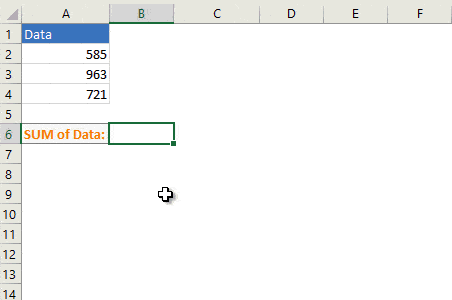### Using Arrow Keys

Here is the whole process:

• Select a cell (using the Arrow Keys in your keyboard) where you want to input the formula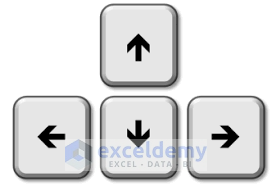• Input an equal (=) sign
• Now navigate the Excel worksheet using the Right, Left, Up, or Down arrow key to reach a cell that you want to enter into the formula.
• Place an operator (+, -, /, *, ^, (), etc.) or one or more Excel functions, or anything you feel necessary to complete the formula
• Select the next cell reference using the above method (Navigate with Arrow keys)
• And continue the process if necessary
• Press Enter when you’re done.

The whole process is shown in the following image.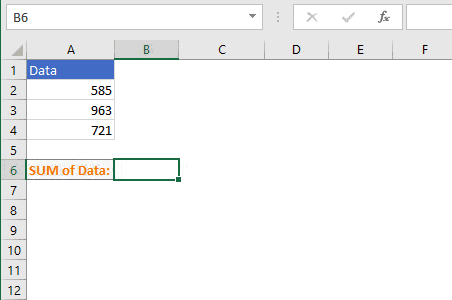## 2) Point and Click Method for Different Worksheets

### Using Mouse Pointer

Here is the whole process:

• Select a cell using your mouse pointer where you want to input the formula
• Input an equal sign (=)
• Now select the worksheet where the data is (using mouse pointer)
• Finally, select the cell references (one by one) that hold data
• Place operators (+, -, /, *, ^, (), etc.) or other necessary components into the formula
• Press Enter when you’re done.

The whole process is shown in the following image.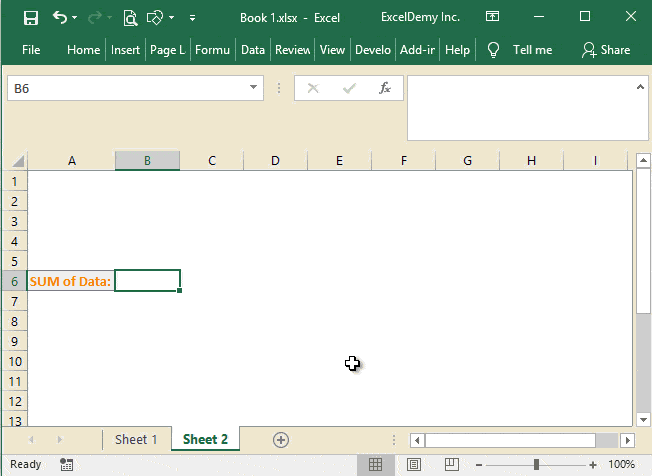### Using Arrow Keys

Here is the whole process:

• Select a cell (using the arrow keys)
• Input an equal sign (=)
• Now navigate to another worksheet (CTRL + Page Up or CTRL + Page Down)
• Now use the Arrow keys to navigate the worksheet > Select a cell or a range of cells (SHIFT + Arrow Keys)
• Press Enter when you’re done.

The whole process is shown in the following image.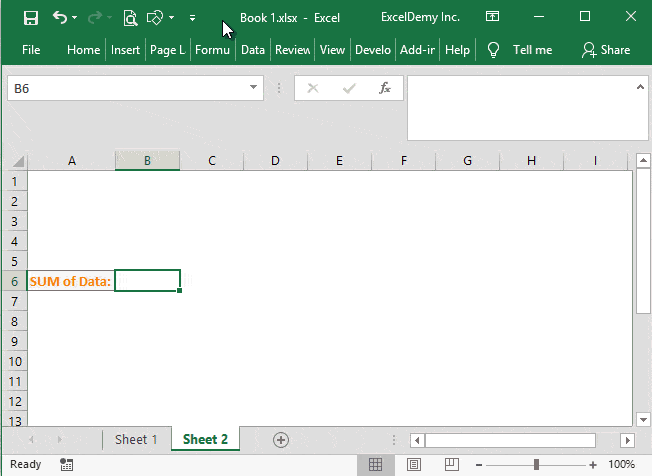## 3) Point and Click Method for Different Workbooks

### Using Mouse Pointer

Here is the whole process:

• Select a cell using your mouse pointer where you want to input the formula
• Input an equal sign (=)
• Now select another workbook > Select a worksheet in the workbook > Select a cell (/cells) where the data is (using mouse pointer)
• Finally, select the cell references (one by one) that hold data
• Place operators (+, -, /, *, ^, (), etc.) or other necessary components into the formula
• Press Enter when you’re done.

The whole process is shown in the following image.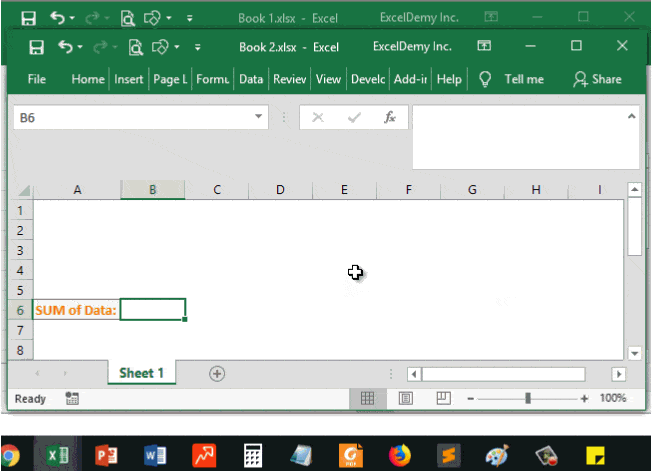### Using Arrow Keys

Here is the whole process:

• Select a cell (using the arrow keys)
• Input an equal sign (=)
• Now navigate to another workbook (using CTRL + Tab key) > Select worksheet in the workbook (CTRL + Page Up or CTRL + Page Down)
• Now use the Arrow keys to navigate the worksheet > Select a cell or a range of cells (SHIFT + Arrow Keys)
• Press Enter when you’re done.

The whole process is shown in the following image.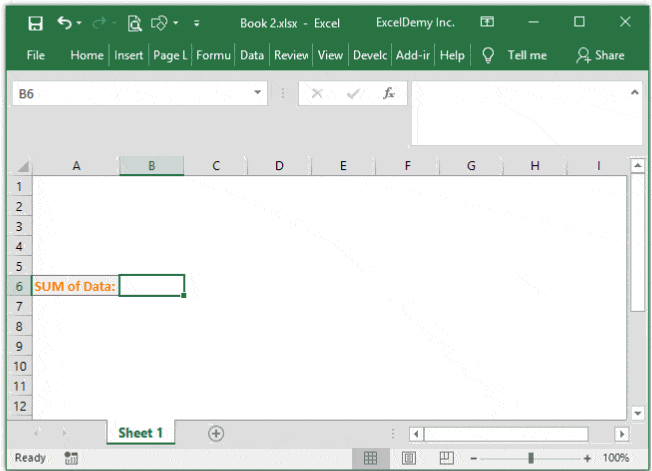## Conclusion

So, to save time and make error-less Excel formulas, use the Point and Click Method. It will also fasten your work. Your comments on this article are highly appreciated.

Hello!
Welcome to my Excel blog! It took me some time to be a fan of Excel. But now I am a die-hard fan of MS Excel. I learn new ways of doing things with Excel and share here. Not only how to guide on Excel, but you will get also topics on Finance, Statistics, Data Analysis, and BI. Stay tuned!

1 Comment
1. ReplyFerreira Feb 19, 2019 at 8:32 PM

Great, thanks